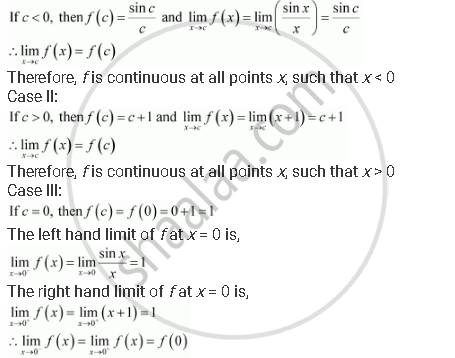= 0) - Mathematics | Shaalaa.com" />= 0) Concept: Concept of Continuity." />= 0)" />
Share

# Find the Points of Discontinuity of F, Where F(X) = {((Sinx)/X, "," If X < 0),(X + 1, "," If X >= 0) - Mathematics

#### Question

Find the points of discontinuity of f, where

f(x) = {((sinx)/x, "," if x < 0),(x + 1, "," if x >= 0):}

#### Solution

The given function f is f(x) = {((sinx)/x, "," if x < 0),(x + 1, "," if x >= 0):}

It is evident that f is defined at all points of the real line.

Let c be a real number.

Case I:Therefore, f is continuous at x = 0

From the above observations, it can be concluded that f is continuous at all points of the real line.

Thus, f has no point of discontinuity.

Is there an error in this question or solution?